StatLect

# Probability space

A probability space is a triple, whereis a sample space,is a sigma-algebra of events andis a probability measure on.## Elements of a probability space

The three building blocks of a probability space can be described as follows:

• the sample spaceis the set of all possible outcomes of a probabilistic experiment;

• the sigma-algebrais the collection of all subsets ofto which we are able/willing to assign probabilities; these subsets are called events;

• the probability measureis a function that associates a probability to each of the events belonging to the sigma-algebra.

## A simple example

Suppose that the probabilistic experiment consists in extracting a ball from an urn containing two balls, one red () and one blue ().

The sample space isA possible sigma-algebra of events iswhereis the empty set.

The four events could be described as follows:

•: nothing happens;

•: either a blue ball or a red ball is extracted;

•: a red ball is extracted;

•: a blue ball is extracted.

A possible probability measureonis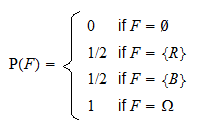Another possibility would be to define the so-called trivial sigma-algebraand specify a probability measureonas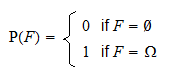## Sample space

How are the three building blocks of a probability space defined?

The first one, the sample space, is a primitive concept, loosely defined as the set of all possible outcomes of the probabilistic experiment.

The other two building blocks are instead defined rigorously, by enumerating the properties (or axioms) that they need to satisfy.

## Sigma-algebra

Letbe a set whose elements are subsets of. Then,is a sigma-algebra if and only if it satisfies the following axioms:

1.;

2. If, then(whereis the complement of, also denoted by)

3. Ifis a countable collection of elements ofand, then.

It is possible to prove that these axioms imply:

•;

• Ifis a countable collection of elements ofand, then.

## Probability measure

Letbe a function that associates a real number to each element of the sigma-algebra. Then,is a probability measure if and only if it satisfies the following axioms:

1. If, then;

2.;

3. Ifis a countable collection of disjoint elements of(i.e.,if), then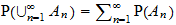.

## General explanation

Why do we impose all these axioms?

Basically, it is for historical reasons and mathematical convenience.

Before Andrey Kolmogorov used the axioms above to define probability, mathematicians had proposed other definitions of probability. Those definitions had several flaws, but they all implied that probability satisfies the axioms above.

By using the axioms as a definition, Kolmogorov was able to develop a theory of probabilities that was logically coherent and without mathematical flaws.

Since then, this definition has been productively used by generations of statisticians, who have built myriads of incredibly useful results upon Kolmogorov's theory.

If it is the first time that you see these axioms, the best strategy to approach them is to memorize them and then convince yourself that they are reasonable. The next sections will help you to do so.

## Explanation of the axioms of a sigma-algebra

Let us start from sigma-algebras.

As we have said, the sigma-algebra contains all the subsets ofto which we wish to assign probabilities.

So, the first thing to keep in mind is that we do not necessarily need to assign a probability to each possible subset of.

Example Suppose that the sample space is the unit interval:Define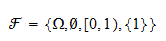You can easily check thatis a sigma-algebra, by verifying that it satisfies the three axioms. It contains very few subsets of. However, if we are interested in the probability of the event, we do not need anything more complicated. Clearly, we can build more complex sigma-algebras. For example, the smallest sigma-algebra that contains the intervalis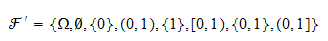A widely used sigma-algebra is the Borel one, which contains all the open subintervals of.

Let us now turn to the three defining axioms.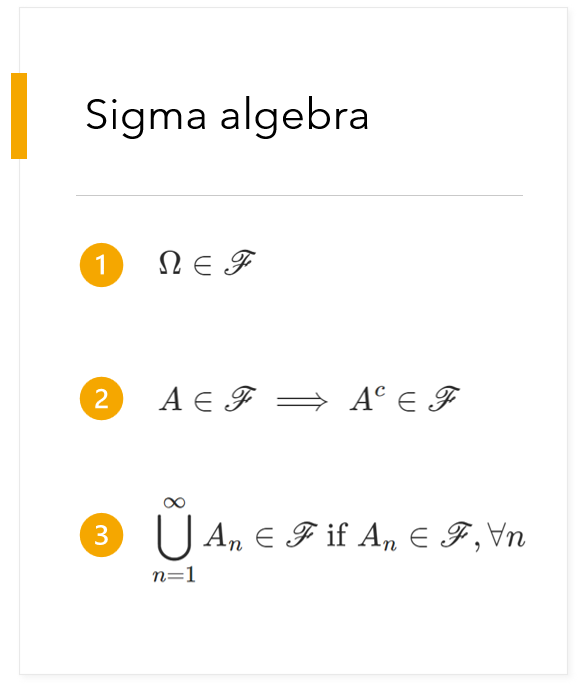Axiom 1 means that we should always be allowed to speak of the trivial event "something will happen".

According to Axiom 2, if we take into consideration the possibility that one of the things in the setwill happen, then we must also consider the alternative possibility that none of the things in the setwill happen.

According to Axiom 3, if we contemplate the possibility that some events will happen (separately for each of them), then we must also be able to assess the possibility that at least one of them will happen (their union).

Axiom 3 is formulated for countable collections of events for mathematical convenience. However, it implies that an analogous property holds for finite collections of events.

In fact, by settingwe obtain## Explanation of the axioms of a probability measure

As we have said, a probability measure attaches a real number (a probability) to each event in the sigma-algebra.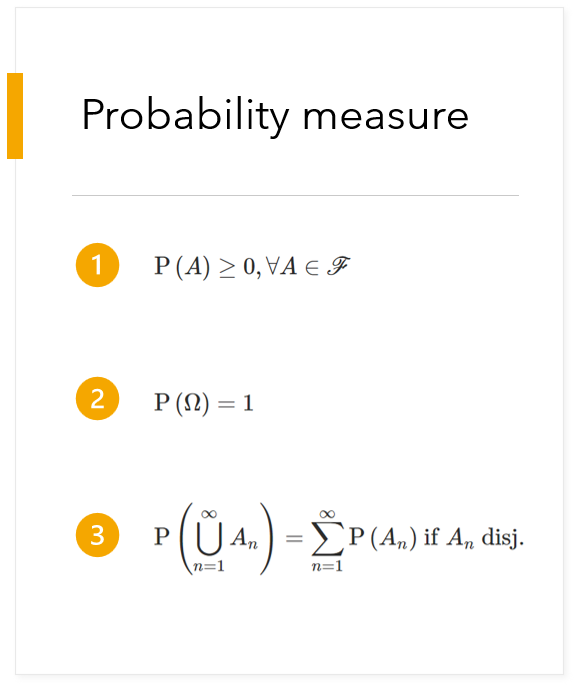Axioms 1 and 2 are established arbitrarily: we simply decide, in line with a centuries-old tradition, that a probability is a positive number smaller than or equal to 1, and that the probability of the sure event is 1.

According to Axiom 3 (called countable additivity), the sum of the probabilities of some disjoint events must be equal to the probability that at least one of those events will happen (their union).

The countable additivity axiom is probably easier to interpret when we setso as to obtain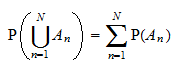which, for, becomes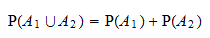## More details and explanations

The lecture on the mathematics of probability contains a more detailed explanation of the concept of probability space and of the properties that must be satisfied by its building blocks.

Previous entry: Probability mass function

Next entry: Random matrix